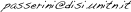# Machine Learning

### General information

 Degree: Master in Computer Science Period: September - December

### Objectives

Provide knowledge of both theoretical and practical aspects of machine learning. Present the main techniques of machine learning and probabilistic reasoning.

### Prerequisites

Linear algebra, probability theory (briefly revised during the course). Boolean algebra, knowledge of a programming language. For a good introduction to linear algebra see: Gilber Strang, Introduction to Linear Algebra, Wellesley-Cambridge Press, 2016.

### Content

Introduction to machine learning: designing a machine learning system, learning settings and tasks, decision trees, k-nearest-neighbour estimation. Mathematical foundations: linear algebra, probability theory, statistical tests. Bayesian decision theory, maximum likelihood and Bayesian parameter estimation. Probabilistic graphical models, inference, parameters and structure learning. Discriminative learning: linear discriminant functions, support vector machines, kernels for vectorial and structured data. Neural networks: representation learning, deep architectures.

### Course Information

 Instructor: Andrea Passerini Email:Teaching assistant: Luca Erculiani Email: luca.erculiani@unitn.it Office hours: Thursday 14:30-15:30 (send email before) Lecture time and place: Wednesday 11:30-13:30 (room a106) Thursday 11:30-13:30 (room a205) Bibliography: R.O. Duda, P.E. Hart and D.G. Stork, Pattern Classification (2nd edition), Wiley-Interscience, 2001. D. Koller and N. Friedman, Probabilistic Graphical Models, The MIT Press, 2009 J.Shawe-Taylor and N. Cristianini, Kernel Methods for Pattern Analysis, Cambridge University Press, 2004. I. Goodfellow, Y. Bengio and A. Courville, Deep Learning, The MIT Press, 2016 (online version available here). Material: Slides and handouts (pdf format) Introduction [slides] [handouts] Decision Trees [slides] [handouts] K-nearest neighbours [slides] [handouts] Linear algebra [slides] [handouts] Probability theory [slides] [handouts] Evaluation [slides] [handouts] Bayesian decision theory [slides] [handouts] Parameter estimation [slides] [handouts] Bayesian Networks [slides] [handouts] Inference in BN [slides] [handouts] Learning BN [slides] [handouts] Naive Bayes [slides] [handouts] Linear discriminant functions [slides] [handouts] Support Vector Machines [slides] [handouts] Non-linear Support Vector Machines [slides] [handouts] Kernel Machines [slides] [handouts] Kernels [slides] [handouts] Deep Networks [slides] [handouts]

### Exams

 Modality: Exercises during course and oral examination Exercises: Bayesian Network lab [slides] [data] [software] Scikit-learn lab [slides] [material] Deep Network lab [slides] [material]